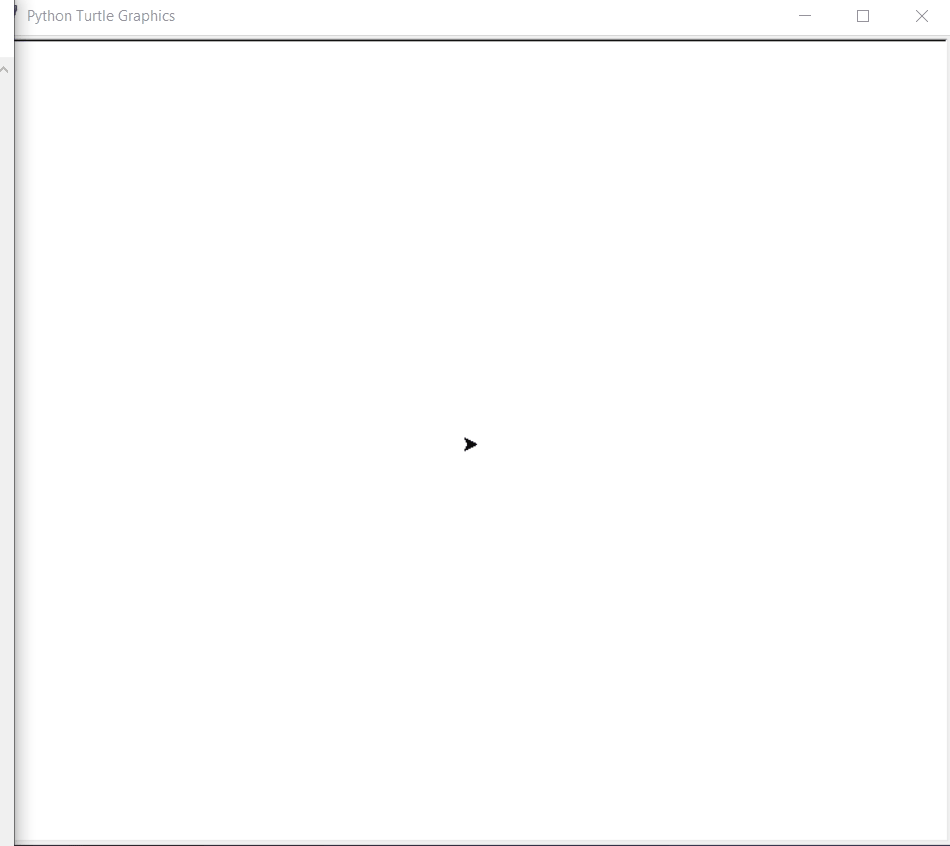Open In App

# Python – Draw Hexagon Using Turtle Graphics

In this article, we will learn how to make a Hexagon using Turtle Graphics in Python. For that lets first know what is Turtle Graphics.

### Turtle graphics

Turtle is a Python feature like a drawing board, which let us command a turtle to draw all over it! We can use many turtle functions which can move the turtle around. Turtle comes in the turtle library.The turtle module can be used in both object-oriented and procedure-oriented ways.

Some of the commonly used methods are:

• forward(length): moves the pen in the forward direction by x unit.
• backward(length): moves the pen in the backward direction by x unit.
• right(angle): rotate the pen in the clockwise direction by an angle x.
• left(angle): rotate the pen in the anticlockwise direction by an angle x.
• penup(): stop drawing of the turtle pen.
• pendown(): start drawing of the turtle pen.

### Approach –

• Define an instance for turtle.
• For a hexagon execute a loop 6 times.
• In every iteration move turtle 90 units forward and move it left 300 degrees.
• This will make up Hexagon .

Below is the python implementation of above approach.

## Python3

 `# import the turtle modules``import` `turtle` `# Start a work Screen``ws ``=` `turtle.Screen()` `# Define a Turtle Instance``geekyTurtle ``=` `turtle.Turtle()` `# executing loop 6 times for 6 sides``for` `i ``in` `range``(``6``):` `    ``# Move forward by 90 units``    ``geekyTurtle.forward(``90``)` `    ``# Turn left the turtle by 300 degrees``    ``geekyTurtle.left(``300``)`

### Output:Turtle Making Hexagon